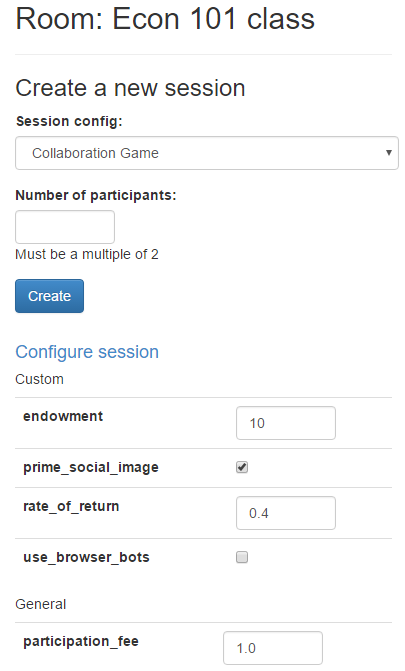# 实验组¶

```def creating_session(subsession):
import random
for player in subsession.get_players():
player.time_pressure = random.choice([True, False])
print('set time_pressure to', player.time_pressure)
```

`creating_session` 会在你点击”create session”按钮后立刻执行，即使应用不在 `app_sequence` 的首位。

## 实验组与多轮次¶

```def creating_session(subsession):
if subsession.round_number == 1:
for player in subsession.get_players():
participant = player.participant
participant.time_pressure = random.choice([True, False])
```

## 平衡实验组¶

```def creating_session(subsession):
import itertools
pressures = itertools.cycle([True, False])
for player in subsession.get_players():
player.time_pressure = next(pressures)
```

## 选择特定实验组进行游戏¶

```SESSION_CONFIGS = [
dict(
name='my_game_primed',
app_sequence=['my_game'],
num_demo_participants=1,
time_pressure=True,
),
dict(
name='my_game_noprime',
app_sequence=['my_game'],
num_demo_participants=1,
time_pressure=False,
),
]
```

```session.config['time_pressure']
```

## 配置会话¶```dict(
name='my_session_config',
display_name='My Session Config',
num_demo_participants=2,
app_sequence=['my_app_1', 'my_app_2'],
num_apples=10
),
```

```dict(
name='my_session_config',
display_name='My Session Config',
num_demo_participants=2,
app_sequence=['my_app_1', 'my_app_2'],
num_apples=10,
doc="""
Edit the 'num_apples' parameter to change the factor by which
contributions to the group are multiplied.
"""
),
```

• 对于一个可配置的参数，它的值必须是数值，布尔类型或字符串。
• 在管理员界面的“Demo”部分，会话是不可配置的。它仅在 “Sessions” 或 “Rooms” 中创建会话时可用。